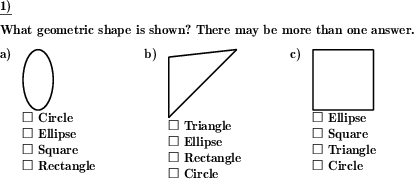Custom math worksheets at your fingertips# Details for problem "Name the 2d geometric shape"

Quickname: 3344

Elementary School, Primary School, Junior High School, Middle School.

## Summary

Name the two dimensional flat geometric shape shown

## Example## Description

A geometric shape is shown. The name has the be determined. Actually, more than one answer may be correct.

For each problem, a single shape is shown. Possible shapes are:

• rectangle
• square
• parallelogram
• rhombus
• trapezoid

Other shapes:

• circle
• ellipse
• triangle

For every shape a selection can be made whether it may occur.

The answer can be requested or presented in the following ways:

• no possible answer is given, the problem is presented as an open question
• multiple possible answers are presented in a multiple choice style. Only one answer is correct.
• multiple possible answers are presented in a multiple choice style. More than one answer may be correct. There is a hint in the problem statement that this may be the case.
• multiple possible answers are presented in a multiple choice style. More than one answer may be correct. There is no hint in the problem statement that this may be the case.

The number of problems is selectable.

Download free printable worksheets for this math problem here. The worksheet contains the problems only, the solution sheet includes the answers. Just click on the respective link.

•Worksheet 1Solution sheet with answers
•Worksheet 2Solution sheet with answers
•Worksheet 3Solution sheet with answers

If you can not see the solution sheets for download, they may be filtered out by an ad blocker that you may have installed. If this is the case, please allow ads for this page and reload the page. The solution sheets will then reappear.

• Do these sample worksheets do not really fit?
• Do you need more math worksheets, with a different level of difficulty?
• Would you like to combine different problems on a worksheet and adjust them to your needs?
• As a teacher, you can put together your own worksheets using the automatically generated math problems provided.
With a free initial credit, you can start creating your own math worksheets in a few minutes.

You can try it for free! Register here, to create custom worksheets now!

## Customization options for this problem

Parameter
Possible values
Number of problems
1, 2, 3, 4, 5, 6
Rectangles
no, rectangle, square, square,rectangle
Triangle
Yes, No
Ellipses
no, ellipse, circle, circle,ellipse
Rhombuses
no, parallelogram, rhombus, parallelogram,rhombus
Trapezoid
Yes, No
open question, multiple choice, mult.choice, mult.correct, mc, no hint on # answers

## dw-Math worksheet templates that do contain this problem

Name
Title
Description
Two-dimensional geometric shapes
Identify, distinguish and name two-dimensional geometric shapes.